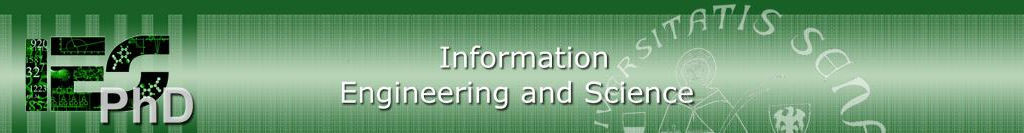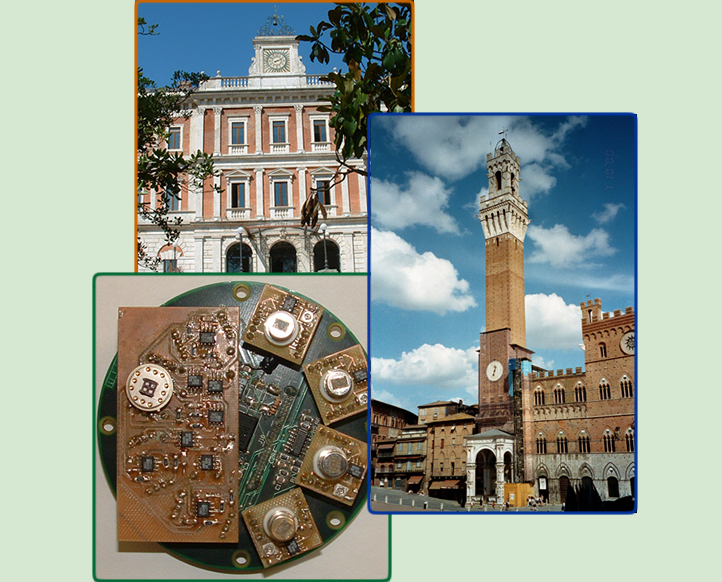Coordinator: Prof. Antonio Vicino University of SienaHome |  DIISM |   | Login Privacy e Cookie policy

InfoHow to applyInformation for PhD   studentsInternal RulesStructurePhD BoardResearch linesLinear Dynamics And Vibration Analysis

 Prof. Monica Malvezzi University of Siena - Dipartimento di Ingegneria dell'Informazione e Scienze Matematiche Course Type Type A/B Calendar April 15-17-21-23-24-28-30 - h. 9.30-12.30 Room Program Objective: Provide an introduction to the analysis of dynamics and vibrations of mechanical systems. After the course, students will be able to choose the more suitable representation for the dynamics of a mechanical system (lumped parameter models, continuous models, FEM) and analyze free and forced vibrations of linear multi-degree of freedom models of mechanical systems and matrix eigenvalue problems. Schedule: Day 1 - Single DOF systems. - Introduction to dynamics and vibration of lumped-parameter models of mechanical systems. - Free and forced vibrations of single DOF systems: main definitions and some examples (accelerometer, seismograph, vehicle suspension). (est. 4h) Day 2 - Multiple DOF system, analysis. - Multiple DOF systems: main definitions and classification, lumped parameter models, continuous models, Finite Element models. Free and forced vibration of linear damped multi-DOF mechanical systems. (est. 4h) Day 3 - Building the mathematical model, discrete systems. - Building a lumped parameter model of a mechanical system: Lagrange's equations for systems of particles and rigid bodies. Linearization of equations of motion. Linear stability analysis of mechanical systems. Inertia, stiffness and damping models. (est. 4h) Day 5 - Building the mathematical model, continuous systems with FEM model. - Continuous systems, introduction - Vibration analysis with Finite Element Analysis: basic theory and applications with Comsol. (est 4h) Day 4 - Building the mathematical model, continuous systems, other methods. - From continuous to lumped parameter models: examples (Holtzer model for torsional vibrations and Myklestad - Prohl model for bending vibrations of rotating shafts). (est. 4h ) Exam: application of one of the modeling tools learned in the course for the analysis of a specific mechanical system.

Courses2020-20212019-20202018-20192017-2018Previous yearsTeachersPoster DayEuropean School of   AntennasPhD Courses UnipiPhD Courses UnipgPhD Students/AlumniPhD studentsAlumniOther PhD programsDip. Ingegneria dell'Informazione e Scienze Matematiche - Via Roma, 56 53100 SIENA - Italy